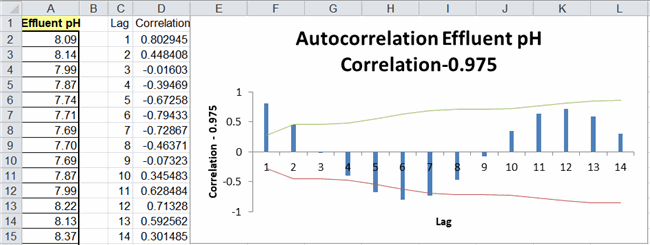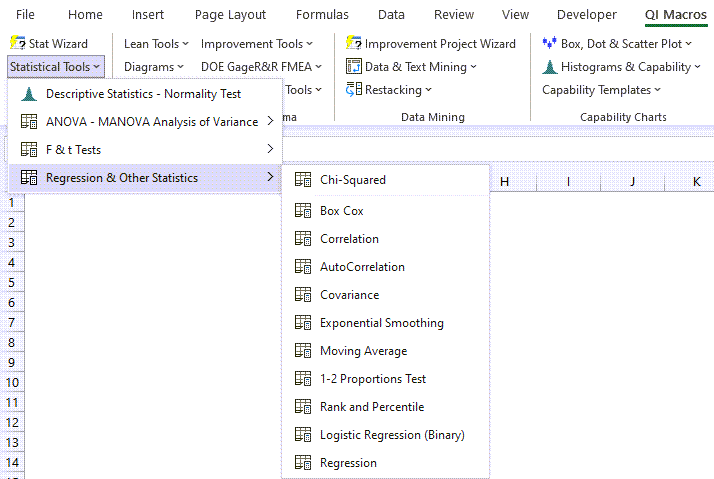# Autocorrelation in Excel using QI Macros

Autocorrelation refers to the degree of correlation between the values of the same variables across different observations in the data.

Imagine seasonal data that rises and falls. The concept of autocorrelation is most often discussed in the context of time series data in which observations occur at different points in time (e.g., air temperature measured on different days of the month). For example, one might expect the air temperature on the 1st day of the month to be more similar to the temperature on the 2nd day compared to the 31st day. If the temperature values that occurred closer together in time are, in fact, more similar than the temperature values that occurred farther apart in time, the data would be autocorrelated.

To understand and explore the autocorrelation between each successive point and lags between points, use the QI Macros autocorrelation function.## How to Conduct Autocorrelation Analysis in Excel using QI Macros

Below is an example of Autocorrelation analysis in Excel using QI Macros.

1. Select one column of data:
2.The sample data shown above is found in QI Macros Help > Open QI Macros Sample Data> XmRChart.xlsx > Autocorrelation tab

3. Next, click on the QI Macros menu and choose Statistical Tools > Regression & Other Statistics > AutoCorrelation:
4.5. Evaluate the Autocorrelation Results:NOTE: Maximum correlations is set to 25.

Correlations for lags:

• If a correlation is outside of the confidence limits (e.g., lag 1 = 0.8), the data is autocorrelated at lag 1.

In this example:

• Lag 1 has a positive autocorrelation (0.8)
• Lag 5 and 6 have a negative autocorrelation (-0.67 and -0.79)

*Functionality introduced in the July 2022 version of QI Macros*

### Why Choose QI Macros Statistical Software for Excel?### Affordable

• Only \$329 USD - less with quantity discounts
• No annual fees
• Free Technical Support### Easy to Use

• Works Right in Excel
• Interprets Results for You
• Accurate Results Without Worry### Proven and Trusted

• 100,000 Users in 80 Countries
• Celebrating 20th Anniversary
• Five Star CNET Rating - Virus Free